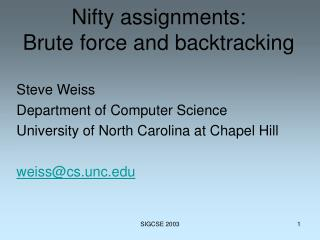# Nifty assignments: Brute force and backtracking - PowerPoint PPT PresentationDownload PresentationNifty assignments: Brute force and backtracking

Nifty assignments: Brute force and backtracking
Download Presentation## Nifty assignments: Brute force and backtracking

- - - - - - - - - - - - - - - - - - - - - - - - - - - E N D - - - - - - - - - - - - - - - - - - - - - - - - - - -
##### Presentation Transcript

1. Nifty assignments:Brute force and backtracking Steve Weiss Department of Computer Science University of North Carolina at Chapel Hill weiss@cs.unc.edu SIGCSE 2003

2. The puzzle SIGCSE 2003

3. SIGCSE 2003

4. Brute force problem solving Generate candidates Filter Solutions Trash SIGCSE 2003

5. Requirements • Candidate set must be finite. • Must be an “Oh yeah!” problem. SIGCSE 2003

6. Example Combination lock 60*60*60 = 216,000 candidates SIGCSE 2003

7. Example SIGCSE 2003

8. Oh no! SIGCSE 2003

9. Oh yeah! SIGCSE 2003

10. Additional restrictions • Solution is a sequence s1, s2,…,sn • Solution length, n, is known (or at least bounded) in advance. • Each si is drawn from a finite pool T. SIGCSE 2003

11. Sequence class • extend(x) Add x to the right end of the sequence. • retract() Remove the rightmost element. • size() How long is the sequence? • … SIGCSE 2003

12. Generating the candidates Classic backtrack algorithm: At decision point, do something new (extend something that hasn’t been added to this sequence at this place before.) Fail: Backtrack: Undo most recent decision (retract). Fail: done SIGCSE 2003

13. Recursive backtrack algorithm(pseudo Java) backtrack(Sequence s) { for each si in T { s.extend(si); if (s.size() == MAX) // Complete sequence display(s); else backtrack(s); s.retract(); } // End of for } // End of backtrack SIGCSE 2003

14. Problem solver backtrack(Sequence s) { for each si in T { s.extend(si); if (s.size() == MAX) // Complete sequence if (s.solution()) display(s); else backtrack(s); s.retract(); } // End of for } // End of backtrack SIGCSE 2003

15. Problems • Too slow, even on very fast machines. • Case study: 8-queens • Example: 8-queens has more than 281,474,976,711,000 candidates. SIGCSE 2003

16. 8-queens • How can you place 8 queens on a chessboard so that no queen threatens any of the others. • Queens can move left, right, up, down, and along both diagonals. SIGCSE 2003

17. Problems • Too slow, even on very fast machines. • Case study: 8-queens • Example: 8-queens has more than 281,474,976,711,000 candidates. SIGCSE 2003

18. Faster! • Reduce size of candidate set. • Example: 8-queens, one per row, has only 16,777,216 candidates. SIGCSE 2003

19. Faster still! • Prune: reject nonviable candidates early, not just when sequence is complete. • Example: 8-queens with pruning looks at about 16,000 partial and complete candidates. SIGCSE 2003

20. Backtrack with pruning backtrack(Sequence s) { for each si in T if (s.okToAdd(si)) // Pruning { s.extend(si); if (s.size() == MAX) // Complete solution display(s); else backtrack(s); s.retract(); } // End of if } // End of backtrack SIGCSE 2003

21. Nifty assignments • Map coloring: Given a map with n regions, and a palate of c colors, how many ways can you color the map so that no regions that share a border are the same color? SIGCSE 2003

22. Nifty assignments • Solution is a sequence of known length (n) where each element is one of the colors. 1 2 4 3 SIGCSE 2003

23. Nifty assignments • 2. Running a maze: How can you get from start to finish legally in a maze? 20 x 20 grid SIGCSE 2003

24. Nifty assignments • Solution is a sequence of unknown length, but bounded by 400, where each element is S, L, or R. SIGCSE 2003

25. Nifty assignments • 3. Making change. • How many ways are there to make \$1.00 with coins. Don’t forget Sacagawea. SIGCSE 2003

26. Nifty assignments • 3. Making change. • Have the coin set be variable. • Exclude the penny. SIGCSE 2003

27. Nifty assignments 4. Unscrambling a word “ptos” == “stop”, “post”, “pots”, ”spot” SIGCSE 2003

28. Nifty assignments 4. Unscrambling a word Requires a dictionary Data structures and efficient search Permutations SIGCSE 2003

29. Nifty assignments • 5. Solving the 9 square problem. • Solution is sequence of length 9 where each element is a different puzzle piece and where the touching edges sum to zero. SIGCSE 2003

30. The puzzle SIGCSE 2003

31. Nifty assignments Challenges: Data structures to store the pieces and the 3 x 3 board. Canonical representation so that solutions don’t appear four times. Pruning nonviable sequences: puzzle piece used more than once. edge rule violation not canonical SIGCSE 2003

32. Nifty assignments Challenges: Algorithm analysis: instrumenting the program to keep track of running time and number of calls to the filter and to the backtrack method. SIGCSE 2003

33. SIGCSE 2003